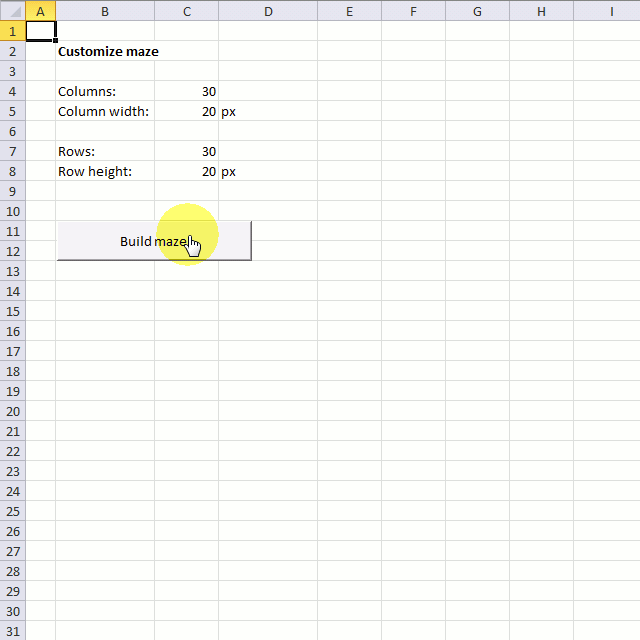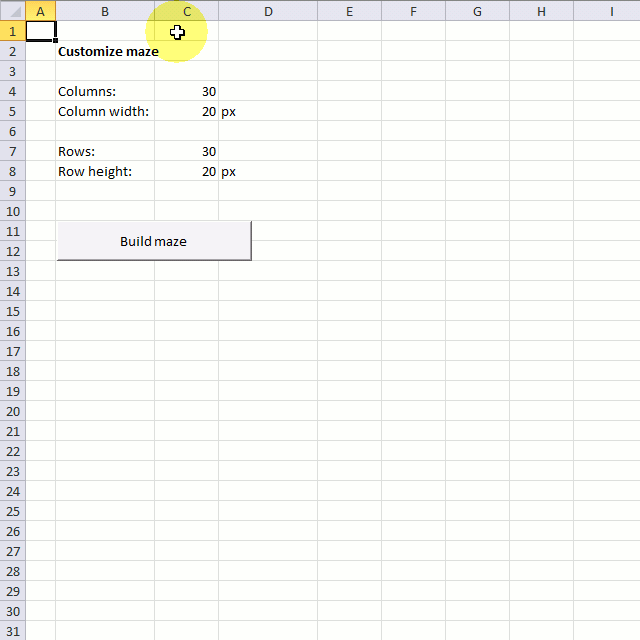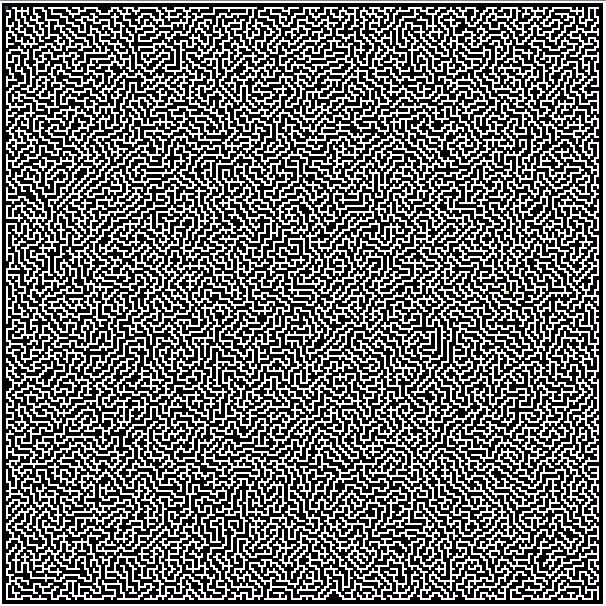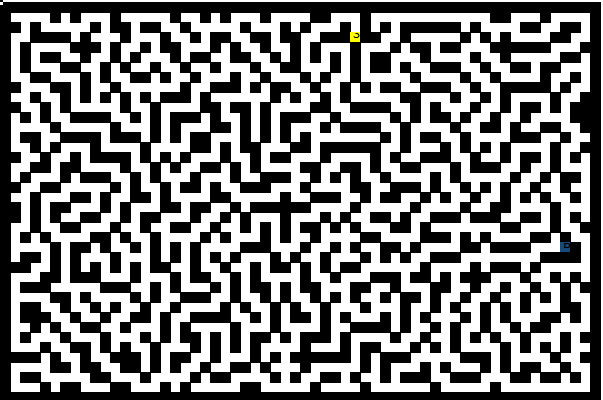Author: Oscar Cronquist Article last updated on November 17, 2020Terry wants to make a different sized maze and I think that is a great idea. Perhaps you remember that I built a maze in excel last week, if you don´t check it out.

This workbook allows you to choose the number of rows and columns you want, as long as it is between 1 and 255. You can also specify the column width and row height.

The following maze has 30 columns and 30 rows.This maze has 200 columns and 200 rows and the column width is 3 px and row height is 3 px.This maze has 60 columns and 40 rows.### VBA Code

```'Name macro
Sub BuildMaze()

'Dimension variables and declare data types
'Variable loc keeps track of location of the current cell
Dim loc() As Variant

'Variable path keeps track of possible cells to go
Dim path(0 To 3)

'visloc keeps track of visited cells, we don't want to move in a direction we already have been to. This keeps the macro from going into an endless loop
Dim visloc() As Variant

'Redimension variable visloc
ReDim visloc(1, 0)

'Disable screen refresh
Application.ScreenUpdating = False

'Select worksheet Maze3
Worksheets("Maze3").Select

'Hide gridlines
ActiveWindow.DisplayGridlines = False

'Select all cells
Cells.Select

'Clear cell range A1:IZ260
Range(Cells(1, 1), Cells(260, 260)) = ""

'The With ... End With statement allows you to write shorter code by referring to an object only once instead of using it with each property.
With Worksheets("Customize maze")

'Redimension array variable loc using values in cell C7 and C4
ReDim loc(0 To .Range("C7").Value, 0 To .Range("C4").Value)

'Change selected cells columnwidths
Selection.ColumnWidth = (.Range("C5").Value / 11.9047619)

'Change selected cells rowheights
Selection.RowHeight = (.Range("C8").Value / (4 / 3))

'Populate cell range with value 1
Range(Cells(2, 2), Cells((.Range("C7").Value + 1), (.Range("C4").Value) + 1)) = 1

'Create a random number based on value in cell C7 and save to variable StartR
StartR = Int(Rnd * (.Range("C7").Value - 2)) + 1

'Create a random number based on value in cell C4 and save to variable StartC
StartC = Int(Rnd * (.Range("C4").Value - 2)) + 1
End With

'Select cell A1
Range("A1").Select

'Enable screen refresh
Application.ScreenUpdating = True

'Disable screen refresh
Application.ScreenUpdating = False

'Save text value "S" to start cell based on variables StartR and StartC
Range("B2").Cells(StartR, StartC) = "S"

'Save number 1 to array variable loc in position based on numbers in variables StartR and StartC
loc(StartR, StartC) = 1

'Save number stored in variable StartR to array variable visloc position row number 0 (zero) and column number 0 (zero)
visloc(0, 0) = StartR

'Save number stored in variable StartC to array variable visloc position row number 1 and column number 1
visloc(1, 0) = StartC

'Save value in cell C7 on worksheet "Customaize maze" to variable Ubr
Ubr = Worksheets("Customize maze").Range("C7").Value

'Save value in cell C7 on worksheet "Customaize maze" to variable Ubr
Ubc = Worksheets("Customize maze").Range("C4").Value

'Do ... Loop Until statement, repeat lines between Do and Loop until a condition is met
Do

'Save 0 (zero) to variable c
c = 0

'For ... Next statement, repeat line 4 times
For i = 0 To 3

'Save number 0 (zero) to array variable path in a position based on variable i
path(i) = 0
Next i

'These If Then statements check if the current cell is inside the boundaries
'If ... then statement
If visloc(0, UBound(visloc, 2)) - 2 >= 1 Then
If loc(visloc(0, UBound(visloc, 2)) - 1, visloc(1, UBound(visloc, 2))) <> 1 And _
loc(visloc(0, UBound(visloc, 2)) - 2, visloc(1, UBound(visloc, 2))) <> 1 And _
loc(visloc(0, UBound(visloc, 2)) - 1, visloc(1, UBound(visloc, 2)) + 1) <> 1 And _
loc(visloc(0, UBound(visloc, 2)) - 1, visloc(1, UBound(visloc, 2)) - 1) <> 1 Then

'Save 1 to variable path position 0 (zero)
path(0) = 1

'Save 1 to variable c
c = 1
End If
End If

'If ... then statement
If visloc(0, UBound(visloc, 2)) + 2 <= Ubr Then
If loc(visloc(0, UBound(visloc, 2)) + 1, visloc(1, UBound(visloc, 2))) <> 1 And _
loc(visloc(0, UBound(visloc, 2)) + 2, visloc(1, UBound(visloc, 2))) <> 1 And _
loc(visloc(0, UBound(visloc, 2)) + 1, visloc(1, UBound(visloc, 2)) + 1) <> 1 And _
loc(visloc(0, UBound(visloc, 2)) + 1, visloc(1, UBound(visloc, 2)) - 1) <> 1 Then

'Save 1 to variable c
c = 1

'Save 1 to variable path position 1
path(1) = 1
End If
End If

'If ... then statement
If visloc(1, UBound(visloc, 2)) - 2 >= 1 Then
If loc(visloc(0, UBound(visloc, 2)), visloc(1, UBound(visloc, 2)) - 1) <> 1 And _
loc(visloc(0, UBound(visloc, 2)), visloc(1, UBound(visloc, 2)) - 2) <> 1 And _
loc(visloc(0, UBound(visloc, 2)) + 1, visloc(1, UBound(visloc, 2)) - 1) <> 1 And _
loc(visloc(0, UBound(visloc, 2)) - 1, visloc(1, UBound(visloc, 2)) - 1) <> 1 Then

'Save 1 to variable c
c = 1

'Save 1 to variable path position 2
path(2) = 1
End If
End If

'If ... then statement
If visloc(1, UBound(visloc, 2)) + 2 <= Ubc Then
If loc(visloc(0, UBound(visloc, 2)), visloc(1, UBound(visloc, 2)) + 1) <> 1 And _
loc(visloc(0, UBound(visloc, 2)), visloc(1, UBound(visloc, 2)) + 2) <> 1 And _
loc(visloc(0, UBound(visloc, 2)) + 1, visloc(1, UBound(visloc, 2)) + 1) <> 1 And _
loc(visloc(0, UBound(visloc, 2)) - 1, visloc(1, UBound(visloc, 2)) + 1) <> 1 Then

'Save 1 to variable c
c = 1

'Save 1 to variable path position 3
path(3) = 1
End If
End If

'Check if c equals 0 (zero)
If c = 0 Then

'Check if Ccount is larger than Tcount
If Ccount > Tcount Then

'Save value in variable Ccount to variable Tcount
Tcount = Ccount

'Save values stored in array variable visloc to variables Er and Ec
Er = visloc(0, UBound(visloc, 2))
Ec = visloc(1, UBound(visloc, 2))
End If

'Subtract 1 with number stored in variable Ccount and save it to Ccount
Ccount = Ccount - 1

'Redimension array variable visloc
ReDim Preserve visloc(UBound(visloc, 1), UBound(visloc, 2) - 1)

'Move here i c is not equal to 0 (zero)
Else

'Save 0 (zero) to variable c
c = 0

'Do Until ... Loop, iterate lines between Do and Loop until a condition is met
Do Until c <> 0

'Save a random whole value between 0 and 3
rrand = Int(Rnd * 4)

'Check if array variable path in position based on value stored in variable rrand is equal to 1, if so, save value in variable rrand plus 1 to variable c
If path(rrand) = 1 Then c = rrand + 1
Loop

'Redimension variable visloc, add a new container
ReDim Preserve visloc(UBound(visloc, 1), UBound(visloc, 2) + 1)

'Select Case statement
'Lines being rund based on value in variable c
Select Case c

'If variable c is equal to 1 then go here
Case 1
visloc(0, UBound(visloc, 2)) = visloc(0, UBound(visloc, 2) - 1) - 1
visloc(1, UBound(visloc, 2)) = visloc(1, UBound(visloc, 2) - 1)

'If variable c is equal to 2 then go here
Case 2
visloc(0, UBound(visloc, 2)) = visloc(0, UBound(visloc, 2) - 1) + 1
visloc(1, UBound(visloc, 2)) = visloc(1, UBound(visloc, 2) - 1)

'If variable c is equal to 3 then go here
Case 3
visloc(0, UBound(visloc, 2)) = visloc(0, UBound(visloc, 2) - 1)
visloc(1, UBound(visloc, 2)) = visloc(1, UBound(visloc, 2) - 1) - 1

'If variable c is equal to 4 then go here
Case 4
visloc(0, UBound(visloc, 2)) = visloc(0, UBound(visloc, 2) - 1)
visloc(1, UBound(visloc, 2)) = visloc(1, UBound(visloc, 2) - 1) + 1
End Select

'Save value in variable Ccount plus 1 to variable Ccount, in other words, add 1 to value in variable Ccount
Ccount = Ccount + 1

'Clear cell range based on value in array variable visloc
Range("B2").Cells(visloc(0, UBound(visloc, 2)), visloc(1, UBound(visloc, 2))) = ""

'Save number 1 to array variable loc in a position based on values in array variable visloc
loc(visloc(0, UBound(visloc, 2)), visloc(1, UBound(visloc, 2))) = 1

'Show changes on screen
'Add another DoEvents to make this macro work in Excel 365 subscription
DoEvents
End If

k = k + 1

'Show changes on screen every 200 based on variable k
If (k / 200) - Int(k / 200) = 0 Then
Application.ScreenUpdating = True
Application.ScreenUpdating = False
End If
Loop Until visloc(0, UBound(visloc, 2)) = StartR And visloc(1, UBound(visloc, 2)) = StartC

'Show changes on screen
Application.ScreenUpdating = True

'Save text value B to end point based on values in variables Er and Ec
Range("B2").Cells(Er, Ec) = "B"

End Sub
```

### Get the Excel fileCustomize-maze.xlsm